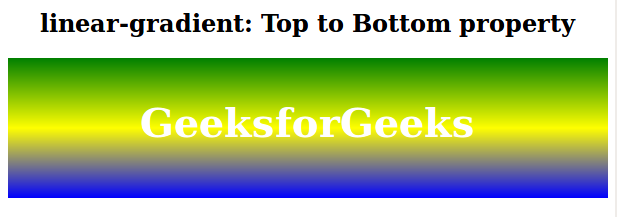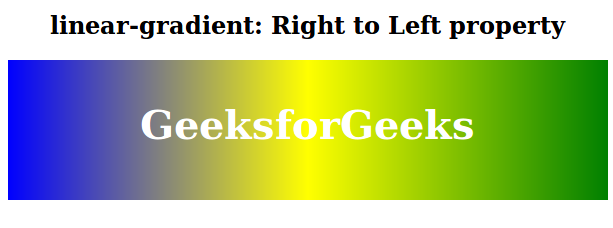Open In App

The linear-gradient() function is an inbuilt function in CSS that is used to set the linear gradient as the background image.

Syntax:

`background-image: linear-gradient( direction, color1, color2, ... )`

Parameters: This function accepts one direction parameter and many color parameters which are listed below:

• direction: This parameter is used to define the starting point and direction along with the gradient effect.
• color1, color2, …: This parameter is used to hold the color value followed by its optional stop position.

The below examples illustrate the linear-gradient() function in CSS:

Example 1: In this example, The .gradient element applies a linear gradient background from green to yellow to blue, with centered text on top.

## html

 ```<``html``>` `<``head``>``    ``<``title``>linear-gradient function``    ``<``style``>``        ``.gradient {``            ``height: 100px;``            ``background-image:``                ``linear-gradient(green, yellow, blue);``            ``Text-align: center;``            ``padding-top: 40px;``            ``font-size: 40px;``            ``color: white;``            ``font-weight: bold;``        ``}` `        ``h2 {``            ``text-align: center;``        ``}``    ````` `<``body``>``    ``<``h2``>linear-gradient: Top to Bottom property``    ``<``div` `class``=``"gradient"``>GeeksforGeeks``` ``

Output:Example 2: The .gradient element applies a linear gradient background from right to left, with colors transitioning from green to yellow to blue.

## html

 ```<``html``>` `<``head``>``    ``<``title``>linear-gradient function``    ``<``style``>``        ``.gradient {``            ``height: 100px;``            ``background-image:``                ``linear-gradient(to left, green, yellow, blue);``            ``Text-align: center;``            ``padding-top: 40px;``            ``font-size: 40px;``            ``color: white;``            ``font-weight: bold;``        ``}` `        ``h2 {``            ``text-align: center;``        ``}``    ````` `<``body``>``    ``<``h2``>``        ``linear-gradient: Right to Left property``    ````    ``<``div` `class``=``"gradient"``>``        ``GeeksforGeeks``    ````` ``

Output:Supported Browser: